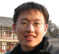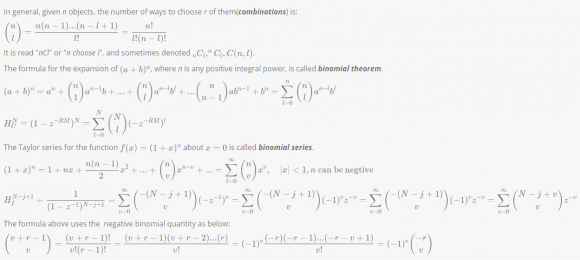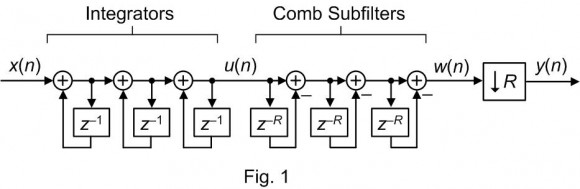Started by 4 months ago4 replieslatest reply 4 months ago250 viewsBefore I have not read Hogenauer's paper, but when I read I am confused about the paper. Could somebody help me?

The denominator of (A2)，

Here |z| = 1, why the second half of the part (A6) can be used here?

hogenauer1981.pdf

[ - ]Hello fpgaplayer.

Look at the center portion of Hogenauer's Eq. (3). That expression is the z-domain transfer function of N integrators cascaded with N comb stages (no decimation, no downsampling).

Now Hogenauer's Eq. (A2) is the z-domain transfer function from the *INPUT* of the jth cascaded integrator to the output of the Nth (last) comb stage. For example, if N = 5 and j = 2 then Eq. (A2) is the transfer function from the input of the second cascaded integrator to the output of the fifth comb stage. That is N-j+1 = 5-2+1 = 4 integrators cascaded with five comb stages.

Notice that if we set j = 1 then Eq. (A-2) becomes equal to the center portion of Hogenauer's Eq. (3).

I don't know how Hogenauer arrived at his Eq. (A-6). However, I believe the factors in Eq. (A-6)'s left bracket are associated with a cascade of N comb stages, and the factors in Eq. (A-6)'s right bracket are associated with a cascade of N-j+1 integrator stages.

[ - ]Hello Pro. Rick Lyons,

Thank you very much for your incredibly fast replay.

It seems that R in Eq.(3) indicates there is a downsampling R, for the Hc(z)=1-z^(-RM).

I have calculated the Eq.(A-6) as below:Yes, the integrator part of Eq.(A6) really a little confuses me with the convergence domain is |z|>1, it seems like there's no Fourier transform for the integrator. Actually, the integrator is not stable, maybe it's more clear from this point of view.

Did I miss anything?

A6calc.png

[ - ]Hi Lyons Zhang (fpgaplayer).

One thing I want to tell you is the following topic: When people
try to learn about CIC decimation (or interpolation) filters it’s
very easy for them to fall into the “trap” of thinking Hogenauer’s
Eq. (3) represents the filters in his paper’s Figure 1 and Figure 2.
I fell into that trap many years ago. Hogenauer’s Eq. (3) does *NOT*
represent the filters in his paper’s Figure 1 and Figure 2! Let me
explain.

Have a look at my Figure 1 below.In my figure there is, indeed, a U(z)/X(z) z-domain transfer
function which is the z-transform of the impulse response at my
network’s u(n) node. And there is a W(z)/X(z) transfer function
which is the z-transform of the impulse response at my network’s
w(n) node.

As taught to me by DSP guru fred harris years ago, there is *NO*
Y(z)/X(z) transfer function describing the relationship of my
network’s decimated y(n) output node to the network’s x(n) input
node. Decimating (after including the downsampling operation) CIC
filters do not have z-domain transfer functions. In my Figure 1
the system whose output is the y(n) node is not “time invariant.”
I discuss this subject at:

https://www.dsprelated.com/showarticle/143.php

So, …Hogenauer’s Eq. (3) is my Figure 1 filter’s W(z)/X(z)
transfer function (prior to the downsampling operation) if we
set his equation’s M = 1 and N = 3.

OK Lyons Zhang, having said all of that, I must say I don’t have the necessary time to slowly plow through your complicated Eq. (A-6) derivation.
But I do compliment you for your algebra skills and your enthusiasm!

I don’t know how to interpret the integrator part of Hogenauer’s
Eq. (6), but I think an integrator doesn’t have a convergent Fourier
transform because its time-domain impulse response is not absolutely
summable. Good Luck.

[ - ]Hello Pro. Rick Lyons，

Thank you for your magnificent fascinating blog https://www.dsprelated.com/showarticle/143.php

Yes, you are right. The system function used to depict the system in the black rectangle of your picture is more accurate.I think it's the root cause that confused me. The z-transform of h[n] is called the system function of the LTI system.

Here the impulse response of the integrator is infinite.

To obtain the DTFT, we always evaluate at z=e^(jω). But the z can evaluate z=e^(a+jw) for z-transform, so I can't arbitrarily say the z-transfer is not applicable for downsampling, but hard to give a precise math description for the convergence domain not include the unit cycle, or for the system there is not convergent DTFT.

Now my understanding is the downsampling is like a modulation operation. Fortunately, the modulation doesn't need LTI space. It just needs vector space.

I may not fully understand the relevant mathematical detailsBut it's enough for me as an application engineer.

Thank you so much again!

[ - ]